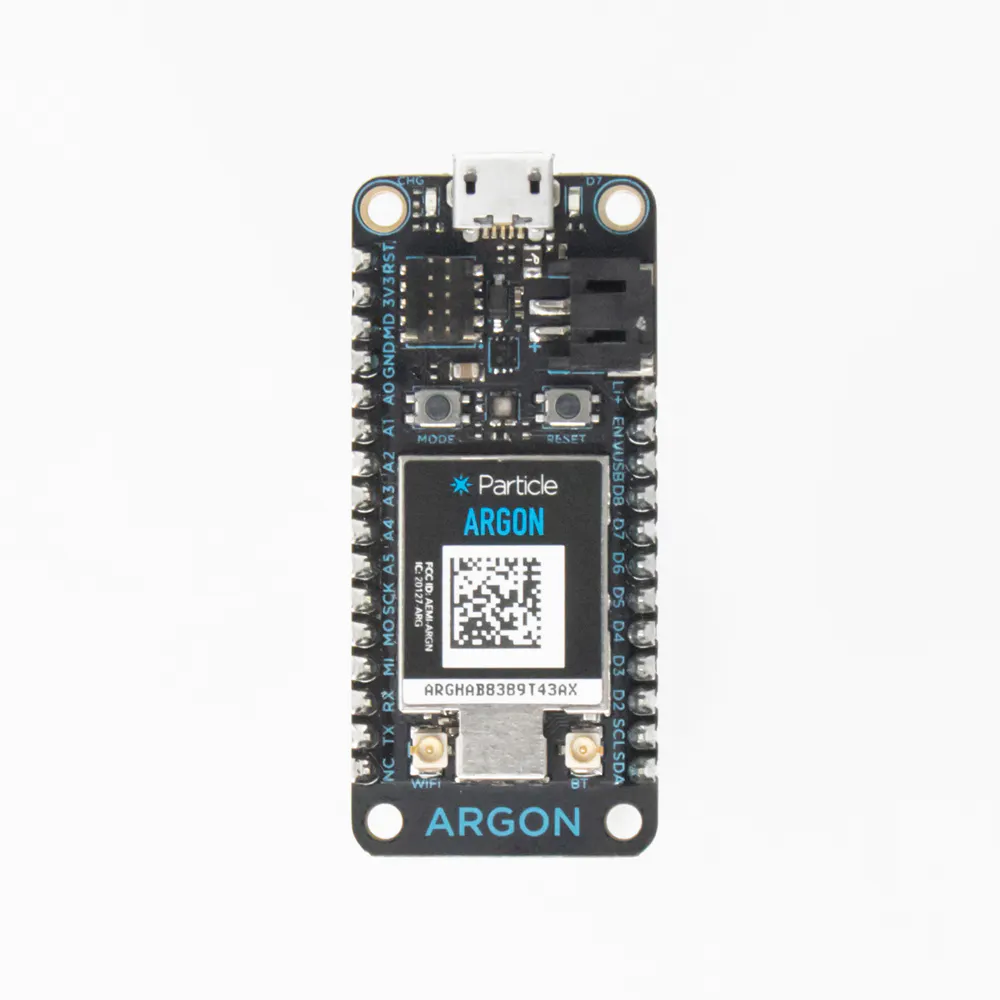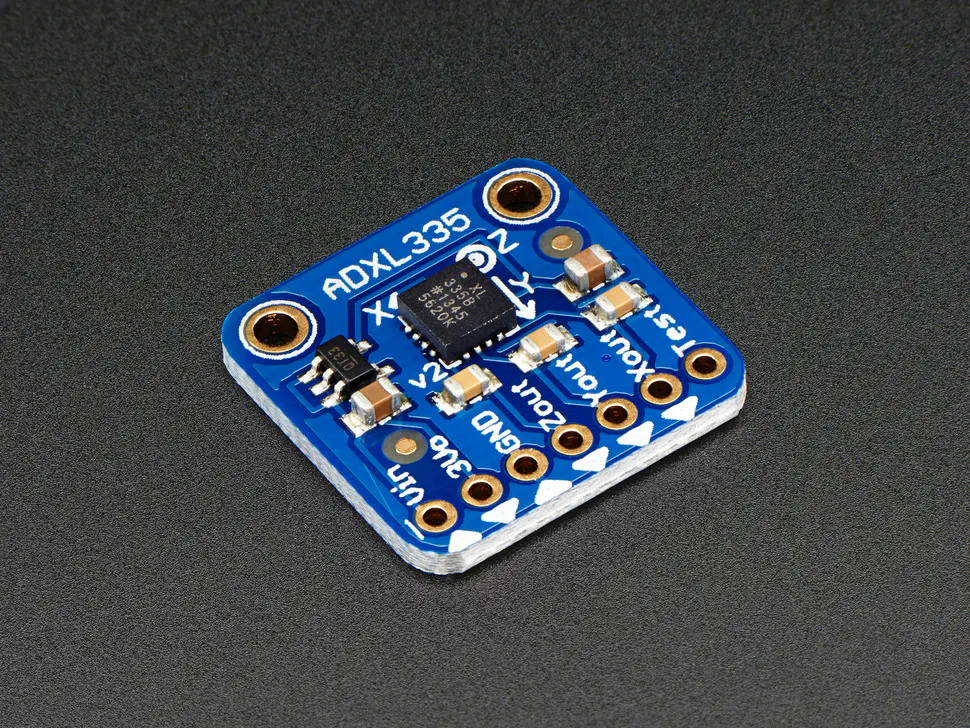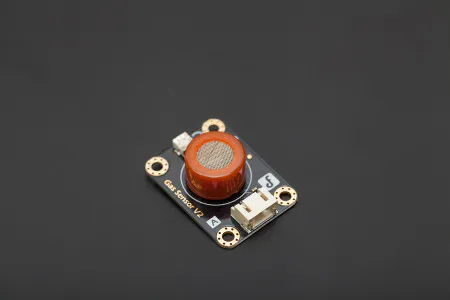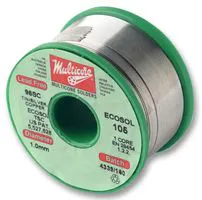Published

Prove to the world that you're a good driver by showing your "Infraction Score, " generated by using argons and sensors.

IntermediateWork in progress15## Things used in this project

### Hardware componentsLED (generic)
×1Jumper wires (generic)
×1Particle Argon
×1
 1W resistors
×1×1×1Ultrasonic Sensor - HC-SR04 (Generic)
×1
 Dupont Connectors
×1
 20Ga Heat-resistant Wire
×1DFRobot Gravity: Analog Alcohol Sensor (MQ3) For Arduino
×1

### Software apps and online servicesParticle Build Web IDE

### Hand tools and fabrication machinesSoldering iron (generic)Solder Flux, Soldering
 Self Solder Heat Seal

## Code

### Accel Sensor

C/C++
This is the code for the accelerometer argon, it transmits the data to the brain argon
```#include <math.h>
const int x_out = A1; /* connect x_out of module to A1 of Particle Photon board */
const int y_out = A2; /* connect y_out of module to A2 of Particle Photon board */
const int z_out = A3; /* connect z_out of module to A3 of Particle Photon board */

void setup() {
Serial.begin(9600);
}

void loop() {
double x_g_value, y_g_value, z_g_value;
double roll, pitch, yaw;
x_adc_value = analogRead(x_out); /* Digital value of voltage on x_out pin */
y_adc_value = analogRead(y_out); /* Digital value of voltage on y_out pin */
z_adc_value = analogRead(z_out); /* Digital value of voltage on z_out pin */
/*
Serial.print("x = ");
Serial.print("\t\t");
Serial.print("y = ");
Serial.print("\t\t");
Serial.print("z = ");
Serial.print("\t\t");
//delay(100);
*/
x_g_value = ( ( ( (double)(x_adc_value * 3.3)/4095) - 1.65 ) / 0.330 ); /* Acceleration in x-direction in g units */
y_g_value = ( ( ( (double)(y_adc_value * 3.3)/4095) - 1.65 ) / 0.330 ); /* Acceleration in y-direction in g units */
z_g_value = ( ( ( (double)(z_adc_value * 3.3)/4095) - 1.80 ) / 0.330 ); /* Acceleration in z-direction in g units */

roll = ( ( (atan2(y_g_value,z_g_value) * 180) / 3.14 ) + 180 ); /* Formula for roll */
pitch = ( ( (atan2(z_g_value,x_g_value) * 180) / 3.14 ) + 180 ); /* Formula for pitch */
//yaw = ( ( (atan2(x_g_value,y_g_value) * 180) / 3.14 ) + 180 ); /* Formula for yaw */
/* Not possible to measure yaw using accelerometer. Gyroscope must be used if yaw is also required */

Serial.print("Roll = ");
Serial.print(roll);
Particle.publish("MEGR3171_IOTGROUP_AccelXgSensor",String(x_g_value),PUBLIC);
//Serial.print("\t");
//Serial.print("Pitch = ");
//Particle.publish("MEGR3171_IOTGROUP_AccelPitchSensor",String(distance),PUBLIC);
//Serial.print(pitch);
//Serial.print("\n\n");
//Particle.publish("MEGR3171_IOTGROUP_AccelPitchSensor",String(distance),PUBLIC);
delay(1500);

}

```

### Sonar Sensor

C/C++
This is the code for the Sonar sensor that transmits data to the brain argon and makes a light blink when the distance is too close.
```// Pin Definitions

#define HCSR04_PIN_TRIG	D2
#define HCSR04_PIN_ECHO	D6

int sound = 0;
int led1 = D0; // Instead of writing D0 over and over again, we'll write led1
// You'll need to wire an LED to this one to see it blink.

int led2 = D7; // Instead of writing D7 over and over again, we'll write led2
// This one is the little blue LED on your board. On the Photon it is next to D7, and on the Core it is next to the USB jack.

void setup() {
// put your setup code here, to run once:
Serial.begin(9600);

pinMode(HCSR04_PIN_TRIG, OUTPUT);
pinMode(HCSR04_PIN_ECHO, INPUT);

//LED Jazz
pinMode(led1, OUTPUT);
pinMode(led2, OUTPUT);

}

void loop() {
// put your main code here, to run repeatedly:
long timeTaken, distance;
digitalWrite(HCSR04_PIN_TRIG, LOW);
delayMicroseconds(2);
digitalWrite(HCSR04_PIN_TRIG, HIGH);
delayMicroseconds(10);
digitalWrite(HCSR04_PIN_TRIG, LOW);
timeTaken = pulseIn(HCSR04_PIN_ECHO, HIGH);//determine distance of wave
distance = (timeTaken/2)/29.1;//using timeTaken calc distance of object

/*determine corressponding leds to light up with respect to the distance
of object*/

if(distance<=60){
Particle.publish("MEGR3171_IOTGROUP_DistanceSensor",String(distance),PUBLIC); //This the command publish data
delay(1500);
digitalWrite(led1, HIGH); //turn on LED
digitalWrite(led2, HIGH);
}
else{
delay(1500);
digitalWrite(led1, LOW);
digitalWrite(led2, LOW);
}
//delay(250);
}

```

### Alcohol Sensor and Brain

C/C++
This is the alcohol sensor code that detects the amount of alcohol in the air. this argon is also the brain and receives data from the other argons and transmits that data to adafruit.
```#define MQ3pin D2

//Particle.publish("MEGR3171_IOTGROUP_AccelXgSensor",String(x_g_value),PUBLIC); //from the accel sensor
//Particle.publish("MEGR3171_IOTGROUP_DistanceSensor",String(distance),PUBLIC); //from the sonar sensor

float sensorValue;  //variable to store sensor value
int score=0; //this will be your drivers score
int alcoholScore= 50; //how much your score will go up if you are drunk

int led = D7; //LED to test if the argons are communicating together
int count=0;

//Distance Stuff
int distance=0; //sonar distance
int disClose= 60; //distance that is too close
int sonarScore=1; //how much score will go up if too close to a car in front of you

//Acceleration Stuff
double acc=0; //accelerometer sensor data
double accMag= 2; //the amount of accel that is too much accel
int accScore=1; //how much score will up if accell to quickly

void setup() {
Serial.println("MQ3 warming up!");
delay(5000); // allow the MQ3 to warm up

/*
for (int i = 1; i <= 10; i++) //this checks your breath for the first ten seconds in setup because this will be checked once
{

if (sensorValue <=500){
Particle.publish("MEGR3171_IOTGROUP_AlcoholSensor",String(sensorValue),PUBLIC);
delay (1500);
}
else{
Particle.publish("MEGR3171_IOTGROUP_AlcoholSensor",String(sensorValue),PUBLIC);
delay (1500);
if (score != 0) //so that it only adds the alcohol once
score= score+alcoholScore;
}

//Particle.publish("MEGR3171_IOTGROUP_AlcoholSensor",String(sensorValue),PUBLIC);
}
*/
pinMode(led, OUTPUT);
digitalWrite(led, LOW);
Particle.subscribe("MEGR3171_IOTGROUP_DistanceSensor", sonar, "e00fce68d0c030a1912bd438");
Particle.subscribe("MEGR3171_IOTGROUP_AccelXgSensor", accel, "e00fce6897f83f6e85dbce03");
Serial.begin(9600); // sets the serial port to 9600

}

void sonar(const char *event, const char *data){ //this is the function that will check the sonar Sensor
distance= String(data).toInt();
if(distance<=disClose){
digitalWrite(led, HIGH); //turn on LED
score= score+ sonarScore;
}
else{
digitalWrite(led, LOW);
}
}
void accel(const char *event, const char *data){ //this is the function that will check the sonar Sensor
acc= String(data).toFloat();
if(acc>=accMag){
digitalWrite(led, HIGH); //turn on LED
score= score+accScore;
}
else{
digitalWrite(led, LOW);
}
}
void loop() {

if (count==0){
for (int i = 1; i <= 10; i++) //this checks your breath for the first ten seconds in setup because this will be checked once
{

if (sensorValue <=500){
Particle.publish("MEGR3171_IOTGROUP_AlcoholSensor",String(sensorValue),PUBLIC);
delay (3000);
}
else{
Particle.publish("MEGR3171_IOTGROUP_AlcoholSensor",String(sensorValue),PUBLIC);
delay (3000);
if (score != 0) //so that it only adds the alcohol once
score= score+alcoholScore;
}
}
count= count +1; //to make sure this only happens once
}

Particle.publish("SonarSensor",String(distance),PUBLIC);
delay(3000);
Particle.publish("AccelSensor",String(acc),PUBLIC);
delay(3000);
//Particle.publish("MEGR3171_IOTGROUP_AlcoholSensor",String(sensorValue),PUBLIC);
//delay (1500);
Particle.publish("MEGR3171_IOTGROUP_Score",String(score),PUBLIC);
delay (3000);

}
```

## Credits

### Elliot Kohut

1 project • 0 followers

### Connor Babcock

1 project • 0 followers

### Michael Burgo

0 projects • 0 followers